Next: Boundary Conditions for and Up: Electrostatics in Dielectric Media Previous: Electrostatics in Dielectric Media

# Polarization

The terrestrial environment is characterized by dielectric media (e.g., air and water) that are, for the most part, electrically neutral, because they are made up of neutral atoms and molecules. However, when the constituent atoms and molecules of such media are placed in an electric field they tend to polarize: that is, to develop electric dipole moments. Suppose that when a given neutral molecule is placed in an electric field,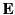, the centre of charge of its constituent electrons (whose total charge is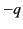) is displaced by a distance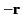with respect to the centre of charge of its nucleus (whose charge is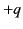). The dipole moment of the molecule is then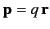. (See Section 2.7.) If there are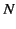such molecules per unit volume then the electric polarization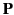(i.e., the dipole moment per unit volume) is given by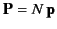. More generally,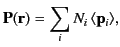(496)

where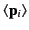is the average dipole moment of the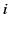th type of molecule in the vicinity of point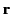, and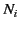is the average number of such molecules per unit volume at.

It is easily demonstrated [e.g., by integrating Equation (201) by parts, and then comparing the result with Equation (162)] that any divergence of the polarization field,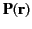, gives rise to a charge density,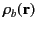, in the medium. In fact,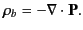(497)

This density is attributable to bound charges (i.e., charges that arise from the polarization of neutral atoms), and is usually distinguished from the charge density,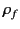, due to free charges, which represents a net surplus or deficit of electrons in the medium. Thus, the total charge density,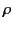, in the medium is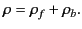(498)

It must be emphasized that both terms on the right-hand side of this equation represent real physical charge. Nevertheless, it is useful to make the distinction between bound and free charges, especially when it comes to working out the energy associated with electric fields in dielectric media.

Gauss' law takes the differential form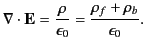(499)

This expression can be rearranged to give(500)

where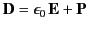(501)

is termed the electric displacement (which should not be confused with dipole moment per unit area--see Section 2.8), and has the same dimensions as(i.e., dipole moment per unit volume). The divergence theorem tells us that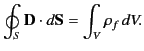(502)

In other words, the flux of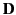out of some closed surface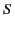is equal to the total free charge enclosed within that surface. Unlike the electric field(which is the force acting on unit charge), or the polarization(which is the dipole moment per unit volume), the electric displacementhas no clear physical meaning. The only reason for introducing this quantity is that it enables us to calculate electric fields in the presence of dielectric materials without first having to know the distribution of bound charges. However, this is only possible if we have a constitutive relation connectingand. It is conventional to assume that the induced polarization,, is directly proportional to the electric field,, so that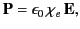(503)

where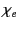is termed the medium's electric susceptibility. It follows that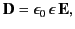(504)

where the dimensionless quantity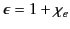(505)

is known as the relative dielectric constant or relative permittivity of the medium. It follows from Equations (501) and (505) that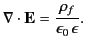(506)

Thus, the electric fields produced by free charges in a dielectric medium are analogous to those produced by the same charges in a vacuum, except that they are reduced by a factor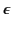. This reduction can be understood in terms of a polarization of the medium's constituent atoms or molecules that produces electric fields in opposition to those of the free charges. One immediate consequence is that the capacitance of a capacitor is increased by a factorif the empty space between the electrodes is filled with a dielectric medium of dielectric constant(assuming that fringing fields can be neglected).

It must be understood that Equations (504)-(507) constitute an approximation that is generally found to hold under terrestrial conditions (provided the electric field-strength does not become too large) when dealing with isotropic media. For anisotropic media (e.g., crystals), Equation (505) generalizes to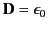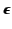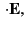(507)

whereis a symmetric second-rank tensor known as the dielectric tensor. For strong electric fields,ceases to vary linearly with. Indeed, for sufficiently strong electric fields, neutral molecules are disrupted, and the medium becomes a plasma.Next: Boundary Conditions for and Up: Electrostatics in Dielectric Media Previous: Electrostatics in Dielectric Media
Richard Fitzpatrick 2014-06-27